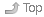# Week 05 Notes for CST8281 - Fall 2011

Fall 2011 - September to December 2011 - Updated 2020-11-12 14:48 EST

# 1 Midterm Test #1 - 15%Index• In class on Friday, October 14. Short answer and multiple choice.
• For full mark credit, read the Test Instructions for important directions on how to enter your answers on the mark-sense forms.
• Calculators are not permitted during the first midterm test. You will benefit from knowing the powers of two from 2^(-4) to 2^16 and the decimal and binary values of the hexadecimal digits from zero to fifteen. Of course you can work them out; however, having at least some of them memorized will make things go faster for you on the test. (Remember that hexadecimal “A” = decimal 10 = binary 1010.)

# 2 Lecture Notes for This WeekIndex## 2.2 From Blackboard Course DocumentsIndexThese documents have restricted distribution and cannot be put on the Course Home Page.

• 01.ppt - Introduction (vonNeumann, prefixes, reciprocal)
• 02.ppt - Data Representation
• omit “Booth’s algorithm” slides 50-52
• ignore slides 63-70 (the “simplified” model)
• slide 72 is wrong: IEEE 754 +0.0 is equal to -0.0
• ignore slides 75-76 (floating-point multiplication)
• use my web pages and assignments instead for floating-point info
• you do not need to know how to do math with sign-magnitude numbers
• you do need to know how to convert them to/from decimal
• you do not need to know how to do math with one’s complement numbers
• you do need to know how to convert them to/from decimal
• you need to know how to do addition with two’s complement numbers
• you also need to know how to convert them to/from decimal
• you do not have to multiply or divide or subtract any binary numbers
• omit most of the math in 2.8 “Error Detection and Correction”
• omit slides 94-100
• omit slides 103-115
• 05.ppt - instruction set architectures
• only read endian-ness on slide 7
• omit the rest of this slide set
• 06.ppt - Memory
• only read memory speeds on slide 7
• omit the rest of this slide set

## 2.4 From the Classroom Whiteboard/ChalkboardIndex• Your in-class notes go here.

• Midterm Test One Date: In class on Friday, October 14. Short answer and multiple choice.

• 000_self_mark.txt - Self-mark of Assignment NN (participation mark)

• Memory speeds (latencies) (06.ppt #7)

• 1ns-2ns - registers
• 3ns-10ns - Level 1 Cache
• 25ns-50ns - Level 2 Cache
• 30ns-90ns - main memory
• 5ms-20ms - fixed hard disk
• Source: [6.ppt] slide 7 (under [Blackboard] Course Content - Slides)

### 2.4.1 Floating PointIndex• Review last week. Look at the 1/10 example program.

• IEEE754 Single Precision: one bit sign, eight bits exponent, 23+1 bits of significand (mantissa). (The leftmost “1.” bit is assumed.)

• Write 1.0 decimal as normalized binary: +1.0 * 2^0

• sign: +
• exponent of 2: 0
• mantissa/significand: 1.0
• as IEEE754 S.P. in binary?
• 8-bit exponent 0 goes in as excess-127, so 0+127 = 127 = 01111111(2)
• What is the next IEEE754 S.P number greater than 1.0?
• Smallest change to significand is from 00000000000000000000000 to 000000000000000000000001 or 2^-23
• multiply by exponent 2^0: 2^-23 * 2^0 = 2^-23
• next number is 1.0 + 2^-23
• Write 8388608 decimal as normalized binary: +1.0 * 2^23

• sign: +
• exponent of 2: 23
• mantissa/significand: 1.0
• As IEEE754 S.P. in binary?
• 8-bit exponent 23 goes in as excess-127, so 23+127 = 150 = 10010110(2)
• What is the next IEEE754 S.P number greater than 8388608?
• Smallest change to significand is from 00000000000000000000000 to 000000000000000000000001 or 2^-23
• multiply by exponent 2^23: 2^-23 * 2^23 = 2^0 = 1
• next number is 8388608 + 1 = 8388609
• See the 02.ppt slide set, but don’t read all the slides.

• Coming up next: Character data

``````Author:
| Ian! D. Allen  -  idallen@idallen.ca  -  Ottawa, Ontario, Canada